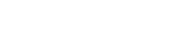# std.strtof

`FLOATstd.strtofSTRINGsINTEGERbase`

Available inall subroutines.

Converts the string `s` to a float value with the given base `base`. The value `base` must be a constant integer expression (variables are not allowed).

The following string formats are supported for finite values:

• Decimal (base 10) floating point syntax. For example, `1.2`, `-1.2e-3`.
• Hexadecimal (base 16) floating point syntax. For example, `0xA.B`, `0xA.Bp-3`.

The syntax for these values corresponds to the syntax for VCL `FLOAT` literals in base 10 and 16 respectively. See VCL Types for details of the `FLOAT` literal syntax.

Supported bases are 0, 10, or 16.

A base of 0 causes the base to be automatically determined from the string format. In this case, a `0x` prefix indicates hex (base 16), and otherwise the base is taken as decimal (base 10).

The syntax is required to match with a corresponding prefix when an explicit base is given. That is, for base 16, the `0x` prefix must be present. Likewise, for base 10, the `0x` prefix must be absent.

Numbers are parsed with a rounding mode of round to nearest with ties away from zero.

In addition to finite values, the following special string formats are supported:

• `NaN`: NaN may be produced by the special format `NaN`. Note that only one NaN representation is produced.
• `inf`, `+inf`, `-inf`: Positive and negative infinities may be produced by the special format `inf` with an optional preceding +/- sign.

The NaN and infinity special formats are case sensitive.

No whitespace is permitted by `std.strtof`.

On error, `fastly.error` is set.

## Errors

If the string could not be parsed, then `fastly.error` will be set to `EPARSENUM`.

If the numeric value would cause overflow, then `fastly.error` will be set to `ERANGE`.

## Example

``declare local var.pi FLOAT;set var.pi = std.strtof(req.http.PI, 10);if (var.pi >= 3.14 && var.pi <= 3.1416) {  set req.http.X-PI = "Close enough";}``

BETA